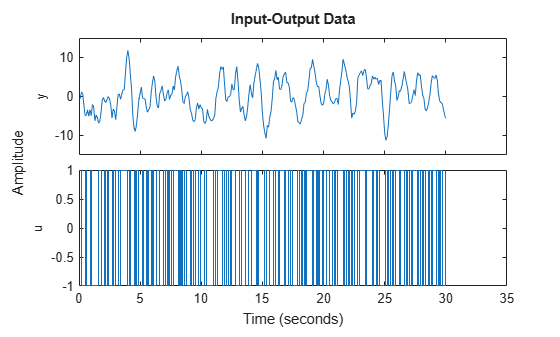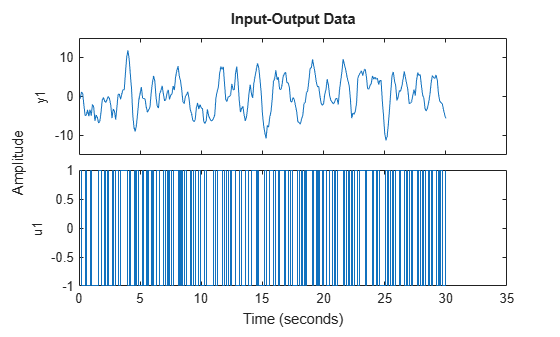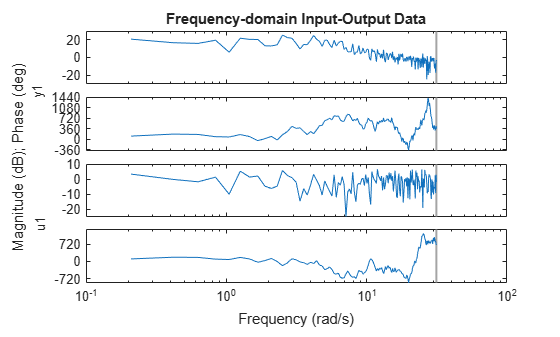# idplot

Plot input and output channels of estimation data

Since R2023a

## Syntax

``idplot(data)``
``idplot(data,LineSpec)``
``idplot(data1,...,dataN)``
``idplot(data1,LineSpec1...,dataN,LineSpecN)``
``idplot(axes_handle,___)``
``sys = idplot(___,Name,Value)``
``idplot(___,plotoptions)``
``h = idplot(___)``

## Description

### Plot Data

example

````idplot(data)` plots the input and output channels of the data `data`. `data` can be a timetable, a comma-separated matrix pair, a single matrix, or an `iddata` object.The function plots the outputs on the top axes and the inputs on the bottom axes. When `data` is a timetable, the software assumes that the last variable is the sole output channel and that the remaining variables are the input channels. If you have data that does not fit this pattern, specify the channels using the `InputName` and `OutputName` name-value arguments.For time-domain data, the input and output signals are plotted as a function of time. The specification for the input intersample behavior (`InputInterSample` option in `dataPlotOptions` when `data` is a timetable or numeric matrix pair, `data.InterSample` when `data` is an `iddata object`) determines whether the input signals are plotted as linearly interpolated curves or as staircase plots. For example, if `data.InterSample = 'zoh'`, the input is piecewise constant between sampling points, and is plotted accordingly.For frequency-domain data, the magnitude and phase of each input and output signal are plotted over the available frequency span.To plot a subset of the data, use subreferencing:`idplot(data(201:300,:))` plots all the variables in the samples 201 to 300 in the timetable object `data`.`idplot(udata(201:300,:),ydata(201:300,:))` plots the samples 201 to 300 in the matrix pair `data`.`idplot(data(201:300))` plots the samples 201 to 300 in the `iddata` object `data`.`idplot(data(201:300,'Altitude',{'Angle_of_attack','Speed'}))` plots the specified samples of the output named `Altitude` and the inputs named `Angle_of_attack` and `Speed`.`idplot(data(:,[3 4],[3:7]))` plots all samples of output channel numbers 3 and 4 and input numbers 3 through 7.```

example

````idplot(data,LineSpec)` specifies the color, line style, and marker symbol for the dataset.```

example

````idplot(data1,...,dataN)` plots multiple datasets. The number of plot axes is determined by the number of unique input and output names among all the datasets.```

example

````idplot(data1,LineSpec1...,dataN,LineSpecN)` specifies the line style, marker type, and color for each dataset. You can specify options for only some data sets. For example, `idplot(data1,data2,'k',data3)` specifies black as the plot color for `data2`.```

### Use Plot Handle to Specify Axes

example

````idplot(axes_handle,___)` plots into the axes with the handle `axes_handle` instead of into the current axes (`gca`). Use this syntax with any of the input argument combinations in the previous syntaxes.```

example

````sys = idplot(___,Name,Value)` incorporates additional options specified by one or more name-value arguments.For example, specify output and input channels using the name-value arguments `OutputName` and `InputName`. Use this syntax when `data` is a timetable and does not follow the default software interpretation of the last variable being the sole output channel and all other channels being input channels.If you specify `'OutputName'` and all the other variables in `data` are input channels, you do not need to specify `'InputName'`.```

### Specify Plot Options

example

````idplot(___,plotoptions)` specifies the plot options.```

### Return Plot Handle

example

````h = idplot(___)` returns the handle to the plot. You can use this handle to customize the plot with `getoptions` and `setoptions`.```

## Examples

collapse all

Load the data in `tt1`, which is a timetable.

`load sdata1 tt1;`

Plot the data.

`idplot(tt1)`The function plots the output on the top axes and the input on the bottom axes.

Plot the first 100 samples.

`idplot(tt1(1:100,:))`Only the first 100 samples appear in the plot.

You can undock and right-click the plot to explore characteristics such as peak and mean values.

Load the data in `umat1` and `ymat1`, which are numeric input and output matrices, and the sample time in `Ts`.

`load sdata1 umat1 ymat1 Ts`

Plot only the input.

`idplot(umat1,[],'Ts',Ts)`Plot only the output.

`idplot([],ymat1,'Ts',Ts)`Plot the input and output together.

`idplot(umat1,ymat1,'Ts',Ts)``load iddata1 z1`

Convert the data to the frequency domain.

`zf = fft(z1);`

Plot the data.

`idplot(zf);`Timetable Data

```load sdata1 tt1 load sdata2 tt2```

Plot both datasets.

`idplot(tt1,tt2)`Because the data sets use the same input and output names, the function plots both data sets together.

Specify unique input and output names.

```tt1.Properties.VariableNames = {'tt1_input' 'tt1_output'}; tt2.Properties.VariableNames = {'tt2_input' 'tt2_output'};```

Plot both datasets.

`idplot(tt1,tt2)`The function plots the data sets separately.

Matrix Data

```load sdata1 umat1 ymat1 load sdata2 umat2 ymat2```

Plot both datasets.

`idplot(umat1,ymat1,umat2,ymat2)`The function plots both data sets together. Because `Ts` is not included in the previous command, the software assumes that the sample time is 1 second.

`iddata` Data

```load iddata1 z1 load iddata2 z2```

Plot both datasets.

`plot(z1,z2)`Because the data sets use the same input and output names, the function plots both data sets together.

Specify unique input and output names.

```z1.InputName = "z1_input"; z2.InputName = "z2_input"; z1.OutputName = "z1_output"; z2.OutputName = "z2_output";```

Plot both datasets.

`plot(z1,z2)`The function plots the data sets separately.

Create a multiexperiment data set.

```load iddata1 z1 load iddata2 z2 zm = merge(z1,z2);```

Plot the data.

```idplot(zm) legend('show')```For multiexperiment data, each experiment is treated as a separate data set. You can right-click the plots to view their characteristics.

Load the timetable `ttsteam`, which contains two input variables and two output variables. View the variable names.

```load steamdata ttsteam ttsteam.Properties.VariableNames```
```ans = 1x4 cell {'Pressure'} {'MagVolt'} {'GenVolt'} {'Speed'} ```

The output variables are `GenVolt` and `Speed`. The input variables are `Pressure` and `Magvolt`.

Try plotting the variables without specifying input and output names.

`idplot(ttsteam)`The software assumes that only the last variable, `'Speed'`, is an output. Use the `'InputName'` and `'OutputName'` name-value arguments to specify the corresponding variables.

```InputName = {"Pressure" "MagVolt"}; OutputName = {"GenVolt" "Speed"}; idplot(ttsteam,'InputName',InputName,'OutputName',OutputName)```In this case, since all the variables in `ttsteam` are either inputs or outputs, you can also specify only the outputs. The software interprets the remaining variables as inputs.

`idplot(ttsteam,'OutputName',OutputName)````load sdata1 umat1 ymat1; load sdata2 umat2 ymat2;```

Specify the line style for both data sets.

`idplot(umat1,ymat1,'y:*',umat2,ymat2,'b')`Create a figure with two subplots and return the handles for each subplot axes in `s`.

```figure % new figure s(1) = subplot(1,2,1); % left subplot s(2) = subplot(1,2,2); % right subplot``````load sdata1 tt1; load sdata2 tt2;```

Create a data plot in each axes using the handles.

`idplot(s(1),tt1)``idplot(s(2),tt2)`Get the handle to your current plot and modify an axis property.

```load iddata1 z1 idplot(z1)```Get the axes handle for the plot.

`ah = gca`
```ah = Axes (u1) with properties: XLim: [0.1000 30] YLim: [-1 1] XScale: 'linear' YScale: 'linear' GridLineStyle: '-' Position: [0.1300 0.1100 0.7750 0.3503] Units: 'normalized' Use GET to show all properties ```

The display shows the properties of the axes handle.

The scale of the x-axis `xScale` is `'linear'`. Change `xScale` to `'log'`.

`ah.XScale = 'log';`The x-axis now displays a log scale.

Configure a time plot.

`opt = dataPlotOptions('time');`

Specify minutes as the time unit of the plot.

`opt.TimeUnits = 'minutes';`

Turn the grid on.

`opt.Grid = 'on';`

Create the plot with the options specified by `opt`.

```load sdata1 umat1 ymat1 Ts idplot(umat1,ymat1,'Ts',Ts,opt);```Create a data plot and return the handle.

```load sdata1 tt1; h = idplot(tt1);```Set the time unit of the plot.

`setoptions(h,'TimeUnits','minutes');`Generate data with two inputs and one output.

`z = iddata(randn(100,1),rand(100,2));`

Configure a time plot.

`opt = iddataPlotOptions('time');`

Plot the data.

`h = plot(z,opt);`Change the orientation of the plots such that all inputs are plotted in one column, and all outputs are in a second column.

```opt.Orientation = 'two-column'; h = plot(z,opt);```Alternatively, use `setoptions`.

`setoptions(h,'Orientation','two-column')`

You can also change the orientation by right-clicking the plot and choosing `Orientation` in the context menu.

## Input Arguments

collapse all

Measured uniformly sampled input/output data, specified as a `timetable`, comma-separated pair of numeric input/output matrices, or `iddata` object. The specification for `data` depends on the data type.

#### Timetable

Specify `data` as a `timetable` that uses a regularly spaced time vector. `tt` contains variables representing input and output channels.

#### Comma-Separated Matrix Pair

Specify `data` as a comma-separated pair of real-valued matrices that contain the input and output time-domain signal values (u,y). Specify the input matrix with the dimensions Ns-by-Nu and the output matrix with the dimensions Ns-by-Ny, where Nu is the number of inputs, Ny is the number of outputs, and Ns is the number of samples.

For time-series data, specify `[]`, y.

#### Data Object

Specify data as an `iddata` object that contains the input and output data. The data can be in the time domain or the frequency domain. It can be a single-channel or multichannel data, and single-experiment or multiexperiment data.

For time-series data (no inputs), specify `data` as an `iddata` object with no inputs.

For more information about working with estimation data types, see Data Domains and Data Types in System Identification Toolbox.

Line style, marker symbol, and color, specified as a character vector. `LineSpec` takes values such as `'b'` and `'b+:'`. For more information, see the `plot` reference page in the MATLAB® documentation. For an example of using `LineSpec`, see Specify Line Style, Marker Symbol, and Color.

Axes handle, specified as a handle, and which is the reference to an `axes` object. Use the `gca` function to get the handle to the current axes, for example, `axes_handle = gca`. For an example of using `axes_handle` to apply a specific set of axes to the current plot, see Specify Axes Handle. For an example of using `gca` to get your plot axes and then modifying the axes properties, see Get and Use Axes Handle.

Plot options, specified as an option set created using `dataPlotOptions`. For an example of using `plotoptions`, see Specify Plot Options.

### Name-Value Arguments

Specify optional pairs of arguments as `Name1=Value1,...,NameN=ValueN`, where `Name` is the argument name and `Value` is the corresponding value. Name-value arguments must appear after other arguments, but the order of the pairs does not matter.

Example: `idplot(data,OutputName=["y2" "y4])` specifies `y2` and `y4` as the output channels in the timetable `data`. Alternatively, you can use `idplot(data,'OutputName',["y2" "y4"])` .

Input channel names for timetable data, specified as a string, a character vector, or an array or cell array of strings or character vectors.

Example: `idplot(data,'InputName',["u1" "u3"])` specifies `u1` and `u3` as the input channels in the timetable `data`.

Output channel names for timetable data, specified as a string, a character vector, or an array or cell array of strings or character vectors.

Example: `idplot(data,'OutputName',["y2" "y4"])` specifies `y2` and `y4` as the output channels in the timetable `data`.

Sample time, specified as the comma-separated pair consisting of `'Ts'` and the sample time in the units specified by `TimeUnit`. When you use matrix-based data (`u`,`y`), you must specify `Ts` if you require a sample time other than the assumed sample time of 1 second.

To obtain the data sample time for a timetable `tt`, use the timetable property `tt.Properties.Timestep`.

Example: `idplot(umat1,ymat1,___,'Ts',0.08)` computes a model with sample time of 0.08 seconds.

Model time units, specified as one of these values:

• `'nanoseconds'`

• `'microseconds'`

• `'milliseconds'`

• `'seconds'`

• `'minutes'`

• `'hours'`

• `'days'`

• `'weeks'`

• `'months'`

• `'years'`

## Output Arguments

collapse all

Plot handle, returned as a scalar or vector. Handles are unique identifiers that you can use to query and modify properties of a specific plot. For an example, see Change Plot Properties Using Handle.

## Tips

Right-clicking the plot opens the context menu, where you can access the following options and plot controls.

OptionDescription and Suboptions
DatasetsView the datasets used in the plot.
Characteristics

Peak Value — View the peak value of the data. This value is useful for transient data.

Mean Value — View the mean value of the data. This value is useful for steady-state data.

Orientation

For data with one input and one output channel:

• Single row — Plot all inputs and outputs in one row.

• Single column— Plot all inputs and outputs in one column.

For data with more than one input or output channel:

• Output row and input row — Plot all outputs in one row and all inputs in a second row.

• Output column and input column — Plot all outputs in one column and all inputs in a second column.

I/O Grouping

Group input and output channels on the plot.

Use this option with datasets with more than one input or output channel.

I/O Selector

Select a subset of the input and output channels to plot. By default, all input and output channels are plotted.

Use this option with data sets with more than one input or output channel.